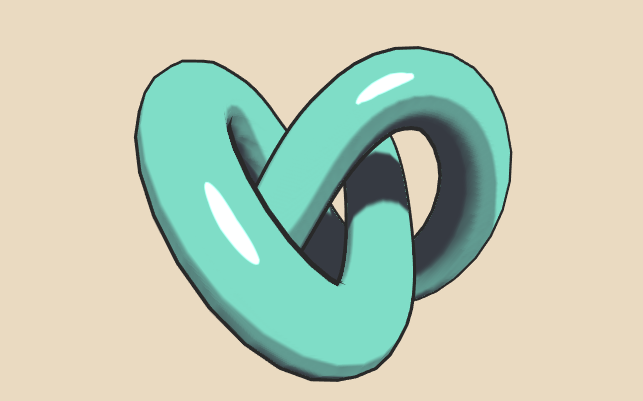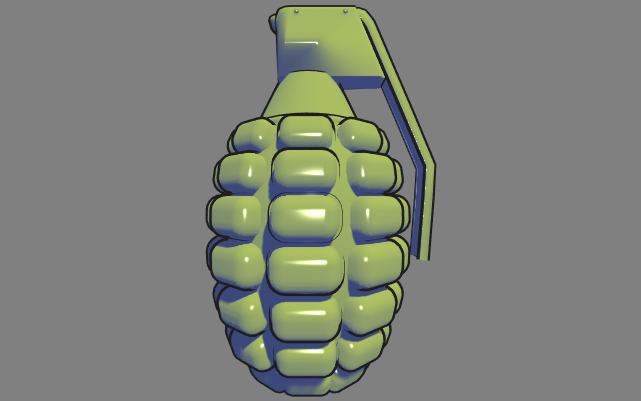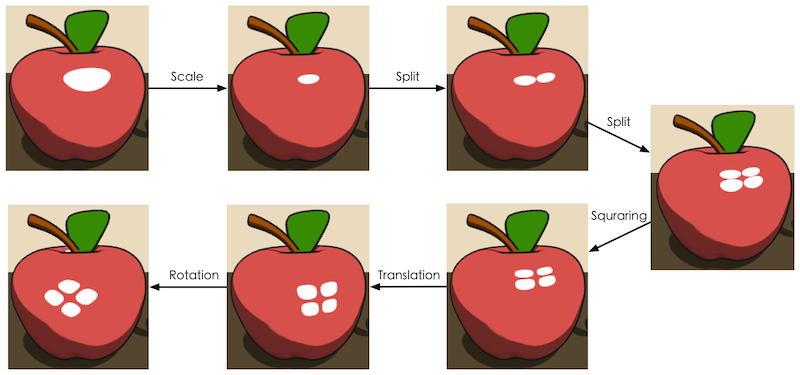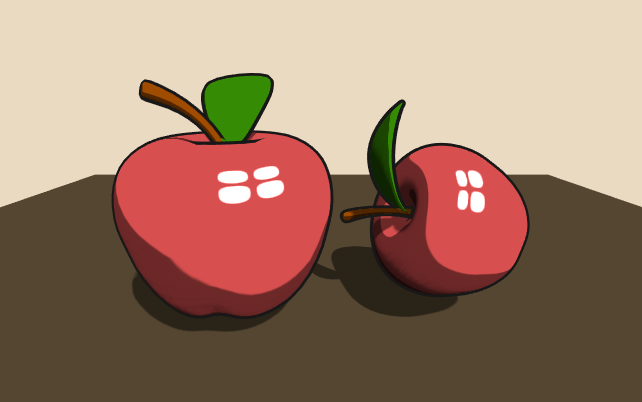# 写在前面

• 一个最常见的包含了卡通风格的漫反射+高光的场景。
• 基于色调的卡通渲染。
• 一种风格化的卡通高光的计算方法。

# 最常见的卡通渲染

## 描边

v2f vert (a2v v) {
v2f o;

float4 pos = mul(UNITY_MATRIX_MV, v.vertex);
float3 normal = mul((float3x3)UNITY_MATRIX_IT_MV, v.normal);
normal.z = -0.5;
pos = pos + float4(normalize(normal), 0) * _Outline;
o.pos = mul(UNITY_MATRIX_P, pos);

return o;
}

float4 frag(v2f i) : SV_Target {
return float4(_OutlineColor.rgb, 1);
}

## 漫反射和高光反射

fixed diff = dot(worldNormal, worldLightDir);
diff = diff * 0.5 + 0.5;
if (diff < _DiffuseSegment.x) {
diff = _DiffuseSegment.x;
} else if (diff < _DiffuseSegment.y) {
diff = _DiffuseSegment.y;
} else if (diff < _DiffuseSegment.z) {
diff = _DiffuseSegment.z;
} else {
diff = _DiffuseSegment.w;
}

fixed3 texColor = tex2D(_MainTex, i.uv).rgb;
fixed3 diffuse = diff * _LightColor0.rgb * _DiffuseColor.rgb * texColor;

fixed w = fwidth(diff) * 2.0;
if (diff < _DiffuseSegment.x + w) {
diff = lerp(_DiffuseSegment.x, _DiffuseSegment.y, smoothstep(_DiffuseSegment.x - w, _DiffuseSegment.x + w, diff));
//  diff = lerp(_DiffuseSegment.x, _DiffuseSegment.y, clamp(0.5 * (diff - _DiffuseSegment.x) / w, 0, 1));
} else if (diff < _DiffuseSegment.y + w) {
diff = lerp(_DiffuseSegment.y, _DiffuseSegment.z, smoothstep(_DiffuseSegment.y - w, _DiffuseSegment.y + w, diff));
//  diff = lerp(_DiffuseSegment.y, _DiffuseSegment.z, clamp(0.5 * (diff - _DiffuseSegment.y) / w, 0, 1));
} else if (diff < _DiffuseSegment.z + w) {
diff = lerp(_DiffuseSegment.z, _DiffuseSegment.w, smoothstep(_DiffuseSegment.z - w, _DiffuseSegment.z + w, diff));
//  diff = lerp(_DiffuseSegment.z, _DiffuseSegment.w, clamp(0.5 * (diff - _DiffuseSegment.z) / w, 0, 1));
} else {
diff = _DiffuseSegment.w;
}

fixed spec = max(0, dot(worldNormal, worldHalfDir));
spec = pow(spec, _Shininess);
w = fwidth(spec);
if (spec < _SpecularSegment + w) {
spec = lerp(0, 1, smoothstep(_SpecularSegment - w, _SpecularSegment + w, spec));
} else {
spec = 1;
}

fixed3 specular = spec * _LightColor0.rgb * _SpecularColor.rgb;# 基于色调的卡通渲染

I=(1+ln2)kcool+(11+ln2)kwarm

kcool=kblue+αkdkwarm=kyellow+βkd# 风格化的卡通高光Hϵ={pS|N(p)H(p)>1ϵ}

H=H+αdu+βdvt(H)=H||H||

// Translation
tangentHalfDir = tangentHalfDir + fixed3(_TranslationX, _TranslationY, 0);
tangentHalfDir = normalize(tangentHalfDir);

// Ratation
float3x3 xRotation = float3x3(1, 0, 0,
0, 1, 0,
0, 0, 1);
tangentHalfDir = mul(zRotation, mul(yRotation, mul(xRotation, tangentHalfDir)));

H=Hδ(Hdu)du,δ[0,1]s(H)=H||H||

// Scale
tangentHalfDir = tangentHalfDir - _ScaleX * tangentHalfDir.x * fixed3(1, 0, 0);
tangentHalfDir = normalize(tangentHalfDir);
tangentHalfDir = tangentHalfDir - _ScaleY * tangentHalfDir.y * fixed3(0, 1, 0);
tangentHalfDir = normalize(tangentHalfDir);

H=Hγ1sgn[(Hdu)]duγ2sgn[(Hdv)]dvspl(H)=H||H||

// Split
fixed signX = 1;
if (tangentHalfDir.x < 0) {
signX = -1;
}
fixed signY = 1;
if (tangentHalfDir.y < 0) {
signY = -1;
}
tangentHalfDir = tangentHalfDir - _SplitX * signX * fixed3(1, 0, 0) - _SplitY * signY * fixed3(0, 1, 0);
tangentHalfDir = normalize(tangentHalfDir);

θ=min(cos1(Hdu),cos1(Hdv)),sqrnom=sin(2θ)n,H=Hσ×sqrnom×((Hdu)du+(Hdv)dv),sqr(H)=H||H||

// Square
float sqrThetaX = acos(tangentHalfDir.x);
float sqrThetaY = acos(tangentHalfDir.y);
fixed sqrnormX = sin(pow(2 * sqrThetaX, _SquareN));
fixed sqrnormY = sin(pow(2 * sqrThetaY, _SquareN));
tangentHalfDir = tangentHalfDir - _SquareScale * (sqrnormX * tangentHalfDir.x * fixed3(1, 0, 0) + sqrnormY * tangentHalfDir.y * fixed3(0, 1, 0));
tangentHalfDir = normalize(tangentHalfDir);

NPR实验室项目中，场景StylizedHighlightsScene实现了上述的渲染方法：# 写在最后

NPR实验室项目目前还包括了一些铅笔风格的实现，有时间再总结吧。希望大家有所收获，就这样~

09-1626
09-011万+
04-242556
05-113235
07-231488
07-231万+
08-061798
08-131621
08-042428
08-3032
04-16
04-24972
07-23324
07-113706
03-201279
08-04400
08-083001

### “相关推荐”对你有帮助么？

•非常没帮助
•没帮助
•一般
•有帮助
•非常有帮助被折叠的  条评论 为什么被折叠?到【灌水乐园】发言¥2 ¥4 ¥6 ¥10 ¥20余额支付 (余额：-- )扫码支付获取中扫码支付点击重新获取扫码支付1.余额是钱包充值的虚拟货币，按照1:1的比例进行支付金额的抵扣。
2.余额无法直接购买下载，可以购买VIP、C币套餐、付费专栏及课程。余额充值